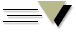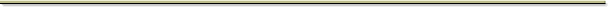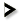## Ars Brevis###11. Questions

Part 11 - Questions

The eleventh part is divided into twelve parts or loci which are disposed and proportioned to questions according to the diversity of their subject matter; now one locus signifies the solution of one question and another locus signifies the solution of another question. This is why we apply questions to the said loci in diverse ways. And we do this in two ways: we put some questions and solve them, and we also put some questions that we do not solve but rather leave it up to the diligent artist to effectively extract the solutions from the parts, or loci, to which we refer the questions, because it is in these parts, or loci, that their solutions are signified.

2. Here we will put and solve only a few questions, for the sake of brevity. Now this Art is abstracted from Ars Magna to give an abridged view of it, so that the intellect can apprehend more meanings through fewer signifiers, and thus become more universal. And by the example of the solutions included or given here, the solutions to other questions can be found, each in its own way.

3. There are twelve loci to which the questions are referred, as said above at the beginning of this chapter. These loci are:

1. the first figure
2. the second figure
3. the third figure
4. the fourth figure
5. the definitions
6. the rules
7. the table
8. evacuating the third figure
9. multiplying the fourth figure
10. mixing principles and rules
11. the nine subjects
12. the 100 forms

Now let us begin with the first locus or part.